# G-function

(diff) ← Older revision | Latest revision (diff) | Newer revision → (diff)

The concept of-functions, not to be confused with the Meijer-functions, was introduced by C.L. Siegel around 1929 [a1], p. 239, in connection with transcendence questions. Consider a Taylor series of the form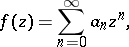where the numbersall belong to a fixed algebraic number field (cf. also Algebraic number; Field)(). Suppose it satisfies the following conditions:

i)satisfies a linear differential equation (cf. also Linear differential operator) with polynomial coefficients;

ii) there exists asuch that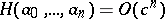. Thenis called a-function. Here, the notation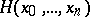stands for the so-called projective height, given by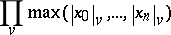for any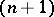-tuple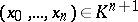. The product is taken over all valuations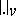of(cf. also Norm on a field). When the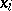are rational numbers,is simply the maximum of the absolute values of thetimes their common denominator. Roughly speaking, Siegel's-functions can be considered as (very interesting) variations on the geometric series, hence the name.

The most common examples are,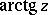, algebraic functions, and the ordinary hypergeometric functions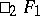with rational parameters (cf. also Hypergeometric function). Siegel introduced them along with their relatives, the-functions (cf.-function), which can be considered as variations on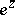.

Although Siegel states some irrationality results for values of-functions at algebraic points, he never published the details of his computations. Subsequent work of A.I. Galoschkin and others showed that there are many more obstacles to get arithmetic results for values of-functions than for-functions. Significant progress was achieved in the 1980{}s, notably by E. Bombieri [a2] and G.V. Chudnovsky [a3]. Much of this progress was related to the properties of-functions themselves and to the question of what-functions really are. If one takes any linear differential equation with coefficients in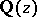, its power series solutions will usually not be-functions. An introduction to these arithmetical problems is given in [a4]. Having a-function solution poses considerable constraints on the arithmetic of a linear differential equation. There exist several conjectures in this direction, the most important being the Bombieri–Dwork conjecture that the differential equation should come from algebraic geometry in a suitable sense. All known-functions actually arise in this way. The converse statement is known to be true, see [a5]. So, statements on the arithmetic nature of values of-functions can also have consequences for problems in algebraic geometry. This point of view was notably adopted by Y. André [a6].

Presently (1996), one can say that Siegel's method and its improvements have not yet given a transcendence proof for a single value of a-function. The fact that such transcendence proofs are a subtle matter was once more confirmed by J. Wolfart's discovery of transcendental-functions having algebraic values at algebraic points [a7]. See also [a8], [a9] for actual computations both in the Archimedean and-adic domain.

How to Cite This Entry:
G-function. Encyclopedia of Mathematics. URL: http://encyclopediaofmath.org/index.php?title=G-function&oldid=15780
This article was adapted from an original article by F. Beukers (originator), which appeared in Encyclopedia of Mathematics - ISBN 1402006098. See original article